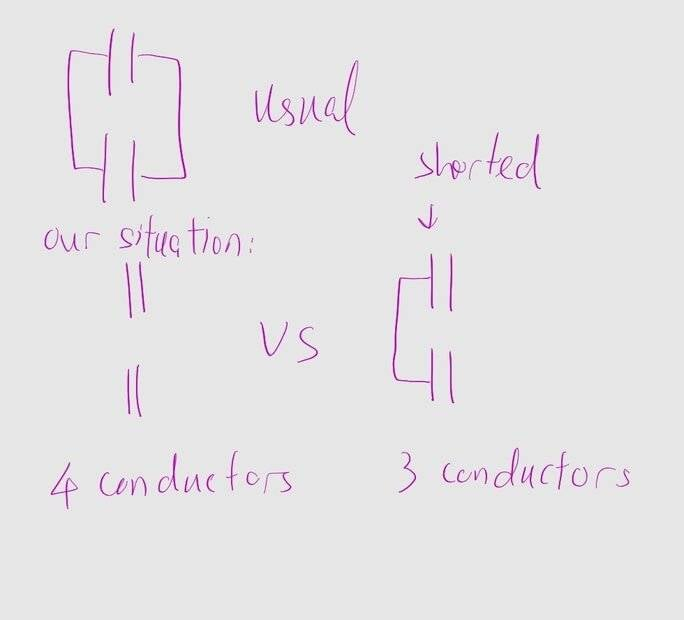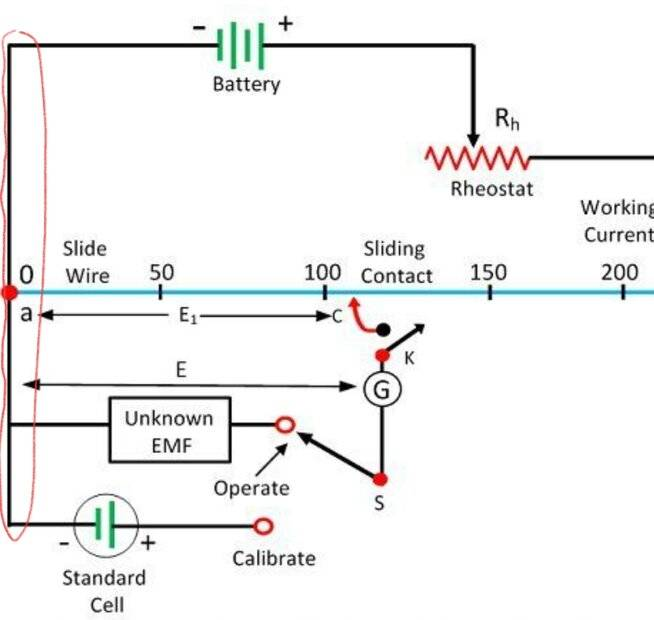# Electric potential and potential difference

• I
• yucheng
In this case, the details of how the external circuit connects to the two capacitors.) (to transition between both)I'm not sure what you mean by "transition between both", but it seems like you are referring to the different cases mentioned above. In summary, connecting only one end of the capacitors will result in a redistribution of charge to equalize the potential, and solving for Maxwell's coefficients may be necessary for more accurate results in non-ideal cases.f

#### yucheng

TL;DR Summary
Electric potential = "absolute potential"

Textbooks usually connect both ends of two capacitors, of different voltages, in parallel. What would happen if we only connect one end of the capacitors? Perhaps we would have to solve for Maxwell's coefficients of potential for these two cases (to transition between both)

Right?

Here's a pictureMy main motivation comes from:

----> https://physics.stackexchange.com/questions/421473/capacitor-connected-to-battery-at-one-end

---> https://www.physicsforums.com/threa...lues-at-battery-terminals.292702/post-2078952
Post #3 "Grounding is a potentially complex and interesting subject rarely dealt with in undergraduate studies."

Do you know of any reference for this?

----> what would happen if we connect batteries in parallel, or that only 1 terminal is shorted. Assume that they have the same potential difference, but that they have different electric potential. Perhaps one realization (potentiometer) (circled red part):----> the problem of using oscilloscopes with floating circuits.

Anywhere I can read further? Thanks!

Last edited:
TL;DR Summary: I'm hoping for resources for further reading, advanced remarks etc

Here's a picture
In order to investigate electric potential, you should let us know how much charges are on the plates.
For an example if there is no charge at all, potential is zero everywhere.

For an example if there is no charge at all, potential is zero everywhere.
With respect, the potential is constant everywhere and we call it zero by convention
TL;DR Summary: I'm hoping for resources for further reading, advanced remarks etc

Electric potential = "absolute potential"

Textbooks usually connect both ends of two capacitors, of different voltages, in parallel. What would happen if we only connect one end of the capacitors? Perhaps we would have to solve for Maxwell's coefficients of potential for these two cases (to transition between both)

Right?

Here's a picture

View attachment 324874

My main motivation comes from:

----> https://physics.stackexchange.com/questions/421473/capacitor-connected-to-battery-at-one-end

---> https://www.physicsforums.com/threa...lues-at-battery-terminals.292702/post-2078952
Post #3 "Grounding is a potentially complex and interesting subject rarely dealt with in undergraduate studies."

Do you know of any reference for this?

----> what would happen if we connect batteries in parallel, or that only 1 terminal is shorted. Assume that they have the same potential difference, but that they have different electric potential. Perhaps one realization (potentiometer) (circled red part):

View attachment 324877

----> the problem of using oscilloscopes with floating circuits.

Anywhere I can read further? Thanks!

The value of the electric potential is defined to within an arbitrary fixed constant and therefore only the differences in potential matter.
The potential of the planet Earth is often defined as zero to make the arithmetic easy.
There is no such thing as an "isolated" object or circuit. One can define the capacitance C of a isolated sphere only by assuming it is electrodynamically connected to the rest of the universe and then $$\Delta V \equiv \Delta Q /C$$ and Q is charge that has been separated from the universe to the object. Usually the rest of the universe is represented by a very large encompassing sphere. For lumped circuit theory the myth of isolation is tacitly assumed sometimes with bad result (as Prof. Walter Lewin marvelously demonstrated )
One could use Maxwell's coefficients but the rest of the universe may need to be included for any meaningful results to obtain. At some level of complexity, the lumped circuit model is probably not the place to start: better to solve Maxwell Equations directly using other approximations as needed.

•yucheng and DaveE
Textbooks usually connect both ends of two capacitors, of different voltages, in parallel.
They won't be different for long. Current will immediately flow to redistribute charges to equalize the two voltages. I don't think you'll see this question too often since it's sort of a trick question with a solution that requires some assumptions about the connecting inductance, oscillations, and energy radiated away as the charge is moved.

What would happen if we only connect one end of the capacitors?
Since you drew this as a lumped element circuit then the answer for the usual case is that you will have the equivalent of a single capacitor with charge Q1+Q2, voltage V1=V2, and capacitance C1+C2. The charge will be redistributed to equalize the potential. For the "3-wire" case, you will have two capacitors in series which is equivalent to a single capacitor with total charge Q1+Q2, voltage V1+V2, and capacitance C1⋅C2/(C1+C2). Of course there will be no current flow, except to equalize the charge on the middle plates (which are eliminated in the single capacitor equivalent).

Perhaps we would have to solve for Maxwell's coefficients of potential for these two cases
Yes, I think so. As @hutchphd said, I don't think you're really asking about a lumped element problem, which is always a huge simplification of the real world. Maxwell's equations are required when the geometric details matter.

•hutchphd
I'm not familiar with the term "Maxwell's Coefficients", Can't find it on Google either could someone explain?

•gleem
Aaah. coefficients of capacitance. Thanks.

•hutchphd
Perhaps I'll just dump some other similar questions here

electricity - Resistor circuit that isn't parallel or series - Physics Stack Exchange
https://physics.stackexchange.com/questions/22252/resistor-circuit-that-isnt-parallel-or-series

electric circuits - Capacitors in Series and Parallel/Dipole - Physics Stack Exchange
https://physics.stackexchange.com/questions/750349/capacitors-in-series-and-parallel-dipole

Connecting 2 capacitors' the leads with opposite polarity
https://images.app.goo.gl/YHWt2G2GkusnCSoX7

electricity - Charged capacitors in series -- but connected at same polarity plates? - Physics Stack Exchange
https://physics.stackexchange.com/q...-series-but-connected-at-same-polarity-plates

electric circuits - Unequal batteries in parallel (theory only & assuming ideal voltage sources) - Physics Stack Exchange
https://physics.stackexchange.com/q...el-theory-only-assuming-ideal-voltage-sources

electricity - Different batteries connected in parallel - Physics Stack Exchange
https://physics.stackexchange.com/questions/64509/different-batteries-connected-in-parallel

electricity - Is a capacitor in an open circuit charged? - Physics Stack Exchange
https://physics.stackexchange.com/questions/103099/is-a-capacitor-in-an-open-circuit-charged